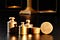# Survey Weights in R

The analysis of survey data usually uses weighting, to make the sample look more like the intended population of the survey.

This article looks at the basic tools in Prof Lumley’s survey R package.

# Weighting with dummy dataWhat weights should be used is a major question in survey research. (Photo: Steen Jepsen/Pixabay)

Weights are often discussed in the analysis of survey data. As an example, if there are slightly fewer women in our sample than we would expect (based on information from the census), researchers may then ‘weigh’ the survey data so the responses for women count for a little more.

I start off by creating some dummy survey data, sampling four variables — meant to resemble age, income, gender and region.

Prof Lumley (University of Auckland) authored the survey package to analyse complex survey data — a major impetus for statistical analysis.

Once we have the survey data, we need to create a survey design object to represent the survey’s design.The svydesign function takes three main arguments:

• ids: this is used to identify clusters, or ~0 or ~1 if there are no clusters;
• data: this is the survey data-set;
• weights: this is the weights used for that data-set, or NULL for unweighted data.

If the survey data comes accompanied with calculated weights, we can input these values using this function.

`dummy_survey_unweighted <- svydesign(ids = ~1,                                      data = dummy_data_df,                                      weights = NULL)`

Next, we could calculate weights based on a known marginal distribution, such as the share of men and women in the population. Another way to is ‘rake’ directly to match that population.

For our dummy data set, we have assumed we have surveyed a population that is 55% women:

`gender_dist <- tibble(gender = c("1", "2"), Freq = nrow(dummy_data_df)*c(0.45, 0.55))dummy_gender_rake <- rake(design = dummy_survey_unweighted,                          sample.margins = list(~gender),                          population.margins = list(gender_dist))`

What is the effect of these survey weights?

`svymean(dummy_data_df,        design = dummy_survey_unweighted)##            mean        SE## age       52.63    1.9634## income 45620.96 2733.5453## gender     1.43    0.0498## region     2.56    0.1175svymean(dummy_data_df,        design = dummy_gender_rake)##              mean        SE## age       53.0653    1.9892## income 45566.0749 2741.9176## gender     1.5500    0.0000## region     2.5645    0.1156`

The mean average age in the dummy data was: 52.6 (for the unweighted data), or 53.0 (for the gender-raked data).